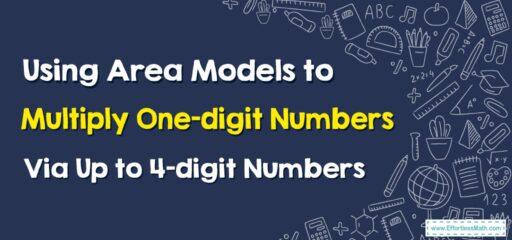# How to Use Area Models to Multiply One-Digit Numbers By Up to 4-digit Numbers

Using an area model can help visualize the multiplication process, especially when dealing with larger numbers.## A Step-by-step Guide to Using Area Models to Multiply One-Digit Numbers By Up to 4-digit Numbers

Here’s a detailed guide on how to use area models to multiply one-digit numbers by up to 4-digit numbers:

Example: Multiply 5 by 1234

### Step 1: Understand the Task

First, identify the numbers you are working with. In this case, the one-digit number is 5 and the four-digit number is 1234.

### Step 2: Create the Area Model

Draw a large rectangle. This rectangle will represent the product of the multiplication.

### Step 3: Divide the Rectangle

Divide the rectangle horizontally into four parts since 1234 is a four-digit number. Each of these sections will represent a digit of 1234.

### Step 4: Label the Rectangle

Along the vertical side (or length) of the rectangle, write the one-digit number (5). Along the horizontal side (or width), write the digits of the four-digit number (1234) above each of the divided sections. In other words, label the first section as 1000, the second section as 200, the third section as 30, and the fourth section as 4. These numbers are the place values of each digit in 1234.

### Step 5: Calculate the Area of Each Section

The area of a rectangle is calculated by multiplying its length by its width. Here, the length is ‘5’ and the widths are ‘1000’, ‘200’, ’30’, and ‘4’. Multiply these out and write the product in each corresponding section. You should get ‘5000’, ‘1000’, ‘150’, and ’20’ respectively.

### Step 6: Sum the Areas

The final step is to add together the products (areas) of all the sections. When you add ‘5000’, ‘1000’, ‘150’, and ’20’ together, you get ‘6170’. This is the product of 5 and 1234.

The area model helps to visualize the multiplication process and makes it easier to grasp, especially when dealing with larger numbers.

### What people say about "How to Use Area Models to Multiply One-Digit Numbers By Up to 4-digit Numbers - Effortless Math: We Help Students Learn to LOVE Mathematics"?

No one replied yet.

X
30% OFF

Limited time only!

Save Over 30%

SAVE $5 It was$16.99 now it is \$11.99